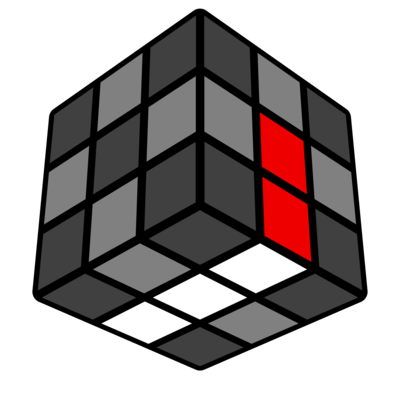# SPL

 SPL methodInformation about the method Proposer(s): Matilda Teixeira Proposed: 2021 Alt Names: Slice Permutation Layers Variants: none No. Steps: 5 No. Algs: 49-300ish Avg Moves: Speed: 65-75 Purpose(s): solving

SPL is a method invented by Matilda Teixeira aka Matilda-Cruzzader25. The creator was inspired to make the method because of the fact that a team in the January 2021 Method Development Competition made one.

## Steps

1. EOSlice: Edges are oriented, while a line consisting of the DL and DR edges is formed.
2. Pseudo F2B: The first two blocks (F2L, but missing the DF and DB edge) are built without regard to Corner Permutation.
3. OCLL: The corners on the last layer are oriented.
4. L6EP: Permute the last six edges. (There is a 50% chance of "parity", where you'll get 2 edges swapped at the end of LSEP. To fix this, you can do a Tperm or Fperm from the CFOP method)
5. CPBL: The corners on U layer and D layer are permuted using one algorithm.

## Variants

Beginners

1. EOSlice: Edges are oriented, while a line consisting of the DL and DR edges is formed.
2. Keyhole Pseudo F2B: The first two blocks (F2L, but missing the DF and DB edge) are built without regard to Corner Permutation and with KeyHole.
3. OCLL: Orient last layer corners.
4. L6EP: Permute the last six edges.
5. CPLL: Permute last layer corners then do a z2 or x2 to permute first layer corners.

COLL

1. EOSlice Edges are oriented, while a line consisting of the DL and DR edges is formed.
2. Pseudo F2B: The first two blocks (F2L, but missing the DF and DB edge) are built without regard to Corner Permutation.
3. COLL: Orient and permute last layer corners.
4. L6EP: Permute the last six edges.
5. CPLL: z2 or x2 to permute first layer corners.

Intermediate

1. EOSlice Edges are oriented, while a line consisting of the DL and DR edges is formed.
2. Pseudo F2B: The first two blocks (F2L, but missing the DF and DB edge) are built without regard to Corner Permutation.
3. OCLL: Orient last layer corners.
4. L6EP: Permute the last six edges.
5. CPLL: Permute last layer corners then do a z2 or x2 to permute first layer corners

Roux AKA Roux-CP

1. Pseudo F2B: The first two blocks (F2L, but missing the DF and DB edge) are solved like in Roux, but without regard to Corner Permutation.
2. OCLL: The corners on the last layer are oriented.
3. CPBL: The corners on U and D are permuted using one algorithm, Edge Permutation can be ignored.
4. LSE: The last six edges are solved like in Roux.

Roux Beginners

1. Keyhole Pseudo F2B: The first two blocks (F2L, but missing the DF and DB edge) are solved like in Roux, but without regard to Corner Permutation.
2. OCLL: The corners on the last layer are oriented.
3. CPLL: Permute last layer corners then do a z2 or x2 to permute first layer corners, Edge Permutation can be ignored.
4. LSE: The last six edges are solved like in Roux.

Roux COLL

1. Pseudo F2B: The first two blocks (F2L, but missing the DF and DB edge) are solved like in Roux, but without regard to Corner Permutation.
2. COLL: The corners on the last layer are oriented and permuted.
3. CPLL: Permute last layer corners then do a z2 or x2 to permute first layer corners, Edge Permutation can be ignored.
4. LSE: The last six edges are solved like in Roux.

Roux Intermidiate

1. Pseudo F2B: The first two blocks (F2L, but missing the DF and DB edge) are solved like in Roux, but without regard to Corner Permutation.
2. OCLL: The corners on the last layer are oriented.
3. CPLL: Permute last layer corners then do a z2 or x2 to permute first layer corners, Edge Permutation can be ignored.
4. LSE: The last six edges are solved like in Roux.

## Pros

• Because CP is ignored, F2L can be solved in fewer moves.

## Cons

• Relatively high movecount (65-75 moves).
• The Edge Orientation step may be hard to get used to for beginners.

## Example solve

Example solve by nuclearaven:

``` Scramble: B' R2 U B2 F2 U L2 D' R2 B2 F2 U' R' U' F2 U2 B L2 F2

x2
L2 F2 U2 F' L' D2 // EOSlice
L' U L U' R U R' // 1st pair
D L U L' D' // 2nd pair
U' R' F U2 R U R' F R // 3rd pair
U' L' U' L U L' U' L U // 4th pair
U R' U2 R U R' U R // OCLL
M2 U' M' U2 M U2 M2 U' M U2 M' // L6EP
x' R' U R' D2 R U' R' D2 R2 // CPBL
```